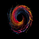# Elastic Volume Weighted Moving Average & Envelope [LazyBear]

13701 lượt xem
13701
Elastic Volume Weighted Moving Average (eVWMA) is a statistical measure using the volume to define the period of the moving average. The eVWMA can be looked at as an approximation to the average price paid per share. Multiplier is usually the number of shares, but it can be approximated using cumulative sum of volume (Enable it via "Use Cumulative Volume" option) or sum of volume over "n" periods.

I have also added an option to draw eVWMA envelope (eVWMA on HLC).

http://christian-fries.de/evwma/PDF/evwm...

List of all my indicators:
```//
// @author LazyBear
// List of all my indicators: https://www.tradingview.com/v/4IneGo8h/
//
study("Elastic Volume Weighted Moving Average [LazyBear]", shorttitle="EVWMA_LB", overlay=true)
length=input(20)
useCV=input(false, type=bool, title="Use Cumulative Volume")
renderBands=input(false, type=bool, title="Draw Envelope")
nbfs = useCV ? cum(volume) : sum(volume, length)
medianSrc=close
calc_evwma(price, length, nb_floating_shares) =>
data = (nz(data) * (nb_floating_shares - volume)/nb_floating_shares) + (volume*price/nb_floating_shares)
data

m=calc_evwma(medianSrc, length, nbfs)
plot(m, color=maroon, linewidth=2, title="evwma")
plot(renderBands ? calc_evwma(high, length, nbfs): na, color=red  , linewidth=2, title="evwma+")
plot(renderBands ? calc_evwma(low, length, nbfs) : na, color=green, linewidth=2, title="evwma-")```
List of my free indicators: http://bit.ly/1LQaPK8
List of my indicators at Appstore: http://blog.tradingview.com/?p=970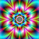Я не пойму значение красной и зеленой линии? Когда входить в сделку?
Phản hồi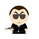Thanks for posting this. The idea of plotting the same function for different sources gives me a ton of ideas.
Phản hồi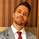This calculation isn't anything new, it is simply the TRUE calculation of the volume weighted moving average. Trading view calculates the VWMA incorrectly as instructed by their own documentation when you search for the vwma() function.

This formula here shows the step-by-step calculation of the VWMA as an incremental/rolling calculation: http://people.ds.cam.ac.uk/fanf2/hermes/doc/antiforgery/stats.pdf, Eq 4-53

U = Un-1 + w / Wn * (x - Un-1)

let Wn = nb_floating_shares (sum of volume (weight) N bars back)
let w = current volume (current weight)
let x = price
let Un-1 = previous MA calculation
let Un = current MA

from line 12 of this script in the calc_evwma function, substituting the above variables:

U = Un-1 * (Wn - w) / Wn + (w * x / Wn)
= Un-1 / Wn * (Wn - w) + w * x / Wn
= Un-1 - Un-1 * w / Wn + w * x / Wn
= Un-1 + w / Wn * (x - Un-1)

This is the exact same formula in the final value in Equation 4-53 above. This is also the same calculation here https://www.tradingsetupsreview.com/volume-weighted-moving-average-vwma/

The Trading View calculation of vwma is wrong, it should be the above. The elastic volume weighted moving average is simply another form of the normal VWMA formula, it is not a new moving average.
Phản hồi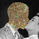mortdiggiddy
@mortdiggiddy, so what you're saying is that this script is the true implementation of the VWMA (using cumulative volume or the sum of the volume over a specified length?) and the code above is the correct implementation of the eVWMA?

Sorry to be extra-dense, but would it be possible for you to script-up the correct implementation of the eVWMA (or whatever it is you've commented above)?

And thank you for sharing those articles. I love when people leave gold in the comments.
Phản hồimortdiggiddy
@mortdiggiddy, Indeed, If you plot the cumulative sum version (All timeSeries from within it's origin price) side by side with VWAP, you will have quite much the same result. Tony Finch's paper is a jewel, it defines and proofs why this kind of incremental rolling could have better computation performance in their AutoRegressive algorithm model. (EWMA for math-statistics, 1-pole Infinite Impulse Response for math-frequentists). Can't remember name of paper for the computational proof for this, but google is always your friend. ;)
Phản hồimortdiggiddy
@mortdiggiddy, I’m new here, so please correct me if I’m wrong.

According to the definition of eVWMA presented in the paper, “Wn” here should be a constant, i.e. total number of shares, that doesn’t have to do with sum of Wi (which would be the total volumes of interest). With Wn != sum of Wi, the equations above do not check out. Hence, it’s more like a volume weighted “cumulative” average (or a special case of VWMA, where all historical volumes are considered), plus a decaying effect on previous trades. Does it make sense?
Phản hồi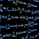Hello, great job. I wish to understand the logic, can you help converting this code into C or Java?
Phản hồiback testing on this on certain setting on one day is incredible, better still with heiken ashi on, just did one stock 91.85%, means 45 wins and 4 losses, every time closed above the evwma, and went up next day higher. So for example... xyz closes on heiken ashi candle above evwma on certain period on one day, you bought on close, next day, first half hour goes higher, so you can get out quick, or sell most shares hold the rest, cause if play right stocks, they often go up days after. I tested a sma, similar setting and found on O (Realty income) where was above the sma line, but dead on, so not above on evwma, and they copied each other closely mind you, so had i followed at the time the sma, would bought and it went down next day. combine this with other things, volume moving averages and bollinger band and regression channel and should be on to 80 to 90% winner. most fascinating. No offense, tried lot of lazy bear indicators and despite high likes, 1000 some of them plus, I'm like no, no good, no matter what settings i use, back testing not that great where as this much better, so congrats.... :)...
Phản hồiThis IS a VERY useful indicator. If you want to trade in a very simple and smart manner, this indicator will help you massively in your trading efforts. Read up on MIDAS is the best thing you can do to under stand how to implement this tool. In a nutshell, only enter a trade when price converges with the line plotted by this indicator. It signifies a "true" value in price. One caveat, you can only use this tool on instruments where volume is measured. So Spot Forex is not compatible ((typical of Forex, not this indicator)), unfortunate for Forex traders, but perhaps that crowd can use a futures equivalent.